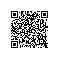# javascript中闭包学习笔记

闭包，从代码的形式上是在函数声明的内部声明另一个函数，从功能上讲，闭包是一种具有状态的函数，函数内的局部变量在函数调用后仍然有效。下面是一个闭包的简单的例子：

function f(){
var cnt =  0;
return function(){ return ++cnt;}
}
var fn=f();     //将函数的返回值赋给变量
fn();
fn();
fn();
上面fn(); 的三次调用输出的分别是 1,2,3，这就说明f()在被调用后其局部变量仍然存在。

闭包的作用：

1、闭包实现访问器

function obj(){
var  _x = 0;

// 返回一个定义了访问器的对象
return  { get x() { return _x;},
set x(v) {_x =v;}
};
}

2、闭包实现信息隐藏

var obj = (function(){
//私有变量
var position = {x:2,y:3};
//私有函数
function sum_internal(a,b){
return Number(a)+Number(b);
}

return{
sum:function(a,b){ return sum_internal(a,b);},
x:position.x
};
}
)();3、使用闭包实现计数器功能的类

function counter_class(i){
var cnt = i || 0;       //设置默认参数
//在此声明私有变量，私有函数
return {
//公有方法
show:function(){ return cnt;},
//return this便于使用方法链
up:function(){cnt++; return this;},
down:function(){cnt--; return this;}
};
}使用钉钉扫一扫加入圈子
+ 订阅